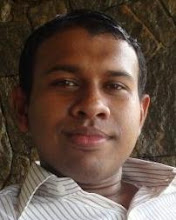## Mufthas Rasikim

### How to draw a cylinder in Java 3D between 2 points

If we go through the documentation of Java 3D, we can see that, there is no direct way to draw a cylinder between 2 Cartesian coordinates. A bond is simply a cylinder. Cylinder can be drawn using ‘Cylinder’ class in Java3D.  Cylinder is defined with a radius and a height. It is a capped cylinder centered at the origin with its central axis aligned the Y-axis. The cylinder constructor accepts radius, height, appearance etc. Here, the bond should be drawn between given two points. In Java3D, a cylinder cannot be drawn between given two points directly. Following steps can be identified to draw a bond between given two points.

1.      Translate the center of the cylinder to the middle point of given two points.
2.      Find the angle of rotation.
3.      Find the axis of rotation
Let’s assume, the end points of the bond are p1 (x1, y1, z1) and p2 (x2, y2, z2). Then the height of the cylinder can be calculated by finding the distance between two points,

Implementing bonds in Java3D

‘Vector3f’ class can be used to represent vectors in Java3D. First of all, the unit vector in the direction of the line connecting two points should be represented by a vector3f object. Cross vector of Y- axis and unit vector in the direction of the line connecting two points should be calculated and assigned to a vector.  Set the angle of rotation about the axis of rotation to an object of ‘AxisAngle4f’. Add this axisangle4f object to an object of ‘Transform3D. Let’s assume this transform3D object as v1.

Translate the center of the cylinder to a new location using an object of ‘Transform3D’. Then multiply this object by transform3D object v1.  Then add the result to an object of ‘TransformGroup’.

Then construct the cylinder with predefined radius, calculated height, custom appearance etc.

Finally, by adding this cylinder to the above transformgroup object, a bond can be constructed.By adding a bond by bond to another new TransformGroup object, the bunch of bonds can be drawn.
Then create an object of ‘BranchGroup’ and add both atoms transformgroup and bond transformgroup to branchgroup object. Finally add the branchgroup object to the ‘SimpleUniverse’ object.

Bond.java

import javax.media.j3d.Appearance;
import javax.media.j3d.Transform3D;
import javax.media.j3d.TransformGroup;
import javax.vecmath.AxisAngle4f;
import javax.vecmath.Vector3f;
import com.sun.j3d.utils.geometry.Cylinder;

public class Bond {

private Vector3f tempVec = new Vector3f();
private Vector3f crossVec = new Vector3f();
private static final Vector3f YAXIS = new Vector3f(0, 1, 0);
private Transform3D tempTrans = new Transform3D();
private Transform3D tempTrans2 = new Transform3D();
private AxisAngle4f tempAA = new AxisAngle4f();
private TransformGroup bondGroup=new TransformGroup();
private TransformGroup bondsGroup=new TransformGroup();

public TransformGroup drawBonds(double[][] coordsBond,Appearance appBond,float radious){

// coordsBond = {x1,y1,z1,x2,y2,z2}
float x,y,z;
double dx,dy,dz,length;

for(int i=0;i

x=(float)(coordsBond[i]+coordsBond[i]);
y=(float)(coordsBond[i]+coordsBond[i]);
z=(float)(coordsBond[i]+coordsBond[i]);
dx=(coordsBond[i]-coordsBond[i]);
dy=(coordsBond[i]-coordsBond[i]);
dz=(coordsBond[i]-coordsBond[i]);

length=Math.sqrt(dx*dx+dy*dy+dz*dz);

tempVec.set((float)dx, (float)dy,(float) dz);

// Find axis of rotation
tempVec.normalize();
crossVec.cross(YAXIS, tempVec);

// Find amount of rotation and put into matrix
tempAA.set(crossVec, (float)Math.acos(YAXIS.dot(tempVec)));
tempTrans.set(tempAA);

// Transform to midpoint between two nodes
tempTrans2.setIdentity();
tempTrans2.setTranslation(new Vector3f(x/2, y/2, z/2));

tempTrans2.mul(tempTrans);

bondGroup=new TransformGroup(tempTrans2);

}//End of for loop
return bondsGroup;
}
}

Dinesh Gamage said...

Oh very interesting. I am willing to use your free open source software in my research as a platform for analyzing

Ranj said...

Hi,

Can you please tell me how to rotate and move a cylinder as if it looks exactly like the hand.
It should be be rotate from one end (which is connected to the body) through out the area.

Thanks and regards,
Ran